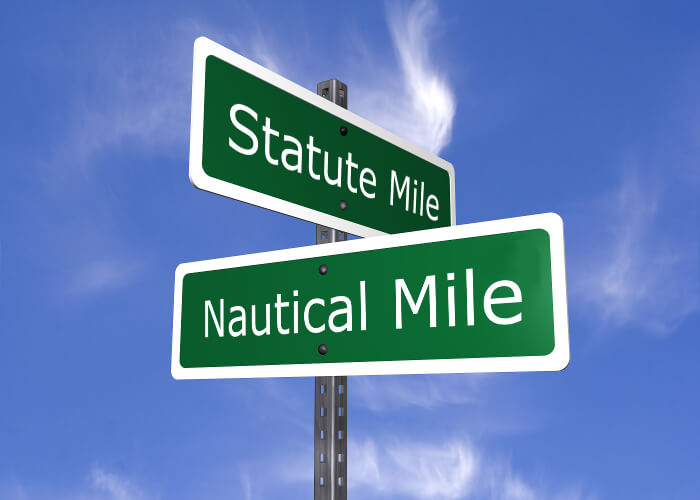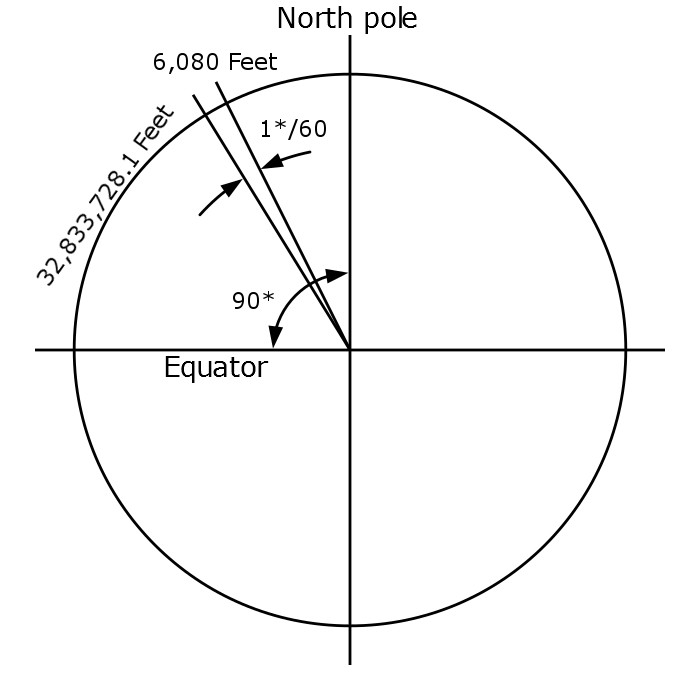Home Learning How Far is A Nautical Mile?

# How Far is A Nautical Mile?

0
5187

If you are in aviation or boating you have heard the terms nautical mile and statute miles more than you probably care to count. But how far is each mile and why are they different?## Statute Mile

Lets start out with a statute mile since most of the world’s English speaking population that doesn’t fly or sail uses this measurement without even knowing it.

1 Statute Mile is the distance of 5280 feet. This decree was created by Queen Elizabeth I in 1593. This standardization of a mile based on feet was to help determine distance while walking on land.

Waaaay back when distance and measuring was not so regulated the people of the world were still looking for a way to communicate size and distance. The ‘foot’ measurement was the size of a full grown man’s foot. However, some data suggests that the actual size referenced was the shoe size rather than the actual foot size.

Fast forward a few decades and centuries and the varying sizes of men’s feet meant that distances and measurements were not consistent. That’s when the British Monarch fixed that problem and standardized units of measure.

Now, how can you determine a foot in the air and on water?

## Nautical Mile

Enter the nautical mile.

I don’t know about you, but the last time I tried to walk on water or walk in the air it didn’t go so well. And before you say, but you could measure your distance over the land, tell me how far over land a boat travels?

So, what was the answer? Math. The universal language of the world.

In basic terms a nautical mile is defined as 1 minute of 1 degree of arc. Wait…but where’s the math?

Nautical Mile = Circumference of the earth (at 45* latitude) / 360* / 60.

Ok smarty pants, where did that come from you ask?

## Mathing the Nautical MileIn 1617 Dutch scientist Snell determined the circumference of the equator to be 24,630 Roman miles (24,024 statute miles). British mathematician Edmund Gunter further refined that by using a circle with same measurements of Snell using the 48* latitude as a guide.

The known math of a circle being 360* is the start of our calculations. 131,334,912.3 feet was the circumference at the 48* latitude using Snell’s calculations. Divide that by 360* and you get 364,819.20 feet between each degree of latitude. From there divide it again by 60 to get 1 minute of distance and you come to 6080.32 feet. Thus, Gunter calculated a nautical mile to be 6,080 feet.

Different mathematicians from around the world used the 45* latitude to make their calculations and came up with 6,076 feet for a nautical mile.

As always, the need for standardization across the world is key in keeping communications consistent. So, in 1929 the international nautical mile was determined to be 6,076 feet with the United States adopting that standardization in 1954.

## Statute vs Nautical Mile

Now that we have the official standards of each mile what is the difference of distance between them?

• 1 Statute Mile = 5280 feet
• 1 Nautical Mile = 6076 feet

6076 / 5280 = 1.15 statute miles per 1 nautical mile.

## Calculating Speed of Each Mile

Now that we know the distance of both miles we can understand how to calculate speed. Which is distance over a period of time.

### Statute Miles

We recognize the speed of miles per hour when it relates to a statute mile. That’s the measurement we use in cars. MPH or miles per hour. 1 MPH is the speed you are going when you take 1 hour to cover the distance of 1 mile.

To put that in perspective, the average human walks about 3.1 MPH. Meaning in one hour of continuous walking the average person will cover 3.1 statute miles.

### Nautical Miles

The unit of measure for speed of a nautical mile is called a Knot. 1 Knot is the speed you are going when you take 1 hour to cover the distance of 1 nautical mile.

This is from the old days of sailing. A rope tied to a plank of wood was thrown over the stern of the ship. Then an hour glass with 30 seconds of sand was used to time how many knots in the rope went overboard. Again, because of math, the distance between each knot on these ropes was 47 feet 3 inches apart.

Let’s put this in perspective, the average human walks about 2.69 Knots. In 1 hour of continuous walking you would cover approximately 2.69 nautical miles.

## Conclusion

The standardization of measurements has made communication and navigation significantly easier over time. Can you imagine trying to give someone directions to your house and one person used the statute mile and another used the nautical mile? You wouldn’t get very far very fast and certainly wouldn’t end up in the destination you were trying to get to if you couldn’t determine how far a nautical mile is.

The statute mile was created based on an arbitrary unit of measure. One day the Queen decided a mile would be so many feet. This is why the metric system makes more sense over the imperial system. At least while trying to determine the measurement of a nautical mile mathematicians tried to make use of some standardization that had practical applications.

Now if only aviation would figure out if they only want to use nautical miles and knots or statute miles and MPH. Think of your airspeed indicator, wind speeds and distances given for certain things. Sometimes the are in MPH, sometimes Knots, sometimes SM (statute miles) and sometimes NM (nautical miles).

What are your thoughts on the standardization of units of measure? Do you think that the FAA and ICAO should stick to one or are you ok bouncing back and forth between both units?